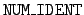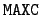Next: Equiprobable bins Up: Structures identifying the data Previous: The array r_react_data   Contents

## The array ident_data

This array gives the basic information for each block of data for each reaction for a target. The dimensions of the array are ident_data(NUM_IDENT, MAXIC), with parameters= 4 and= 400. This array is reset for each new target. Its second index ranges over the reactions C and over the types of data I_number for the target. For each reaction and for each value of I_number the first index identifies the following items.

ident_data(1, I_C_count):
The identifier I_number for the type of data. See Section 2.4.

ident_data(2, I_C_count):
Identifier yo for the first secondary particle. See Section 2.1.

ident_data(3, I_C_count):
The data offset iifwa. Specifically, the location of this data block is given in nhead(iifwa). (The structure of nhead is defined in Section 3.2.3.)

ident_data(4, I_C_count):
The interpolation type to be used on the data. The values used are 0 for linear-linear interpolation and 5 for log-log interpolation.